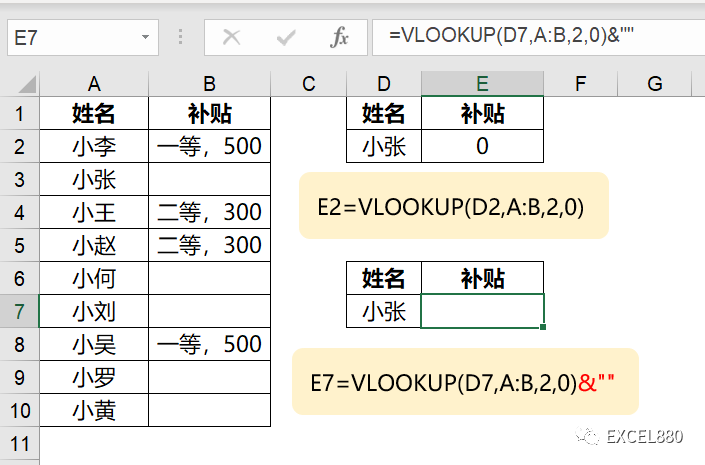# 一组Excel常见疑难杂症实战案例解析

76 次浏览

Excel880笔记：FIND查找时要注意包含关系

=COUNT(FIND(“张君”,B2:B7))

=COUNT(FIND(“,张君,”,”,”&B2:B7&”,”))Excel880笔记：INT向下取整为最近的整数，注意对象是负数的情况

=INT(A3)

-3.14向下最近的整数是-4，而不是-3.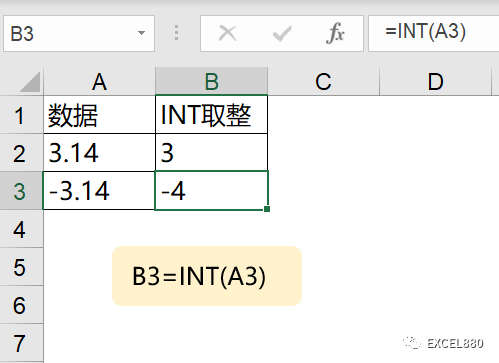Excel880笔记：UNIQUE除了去除重复，还可以提取只出现一次的数据。

UNIQUE第三参数设置为TRUE,返回只出现一次的项。

=UNIQUE(C2:C15,,TRUE)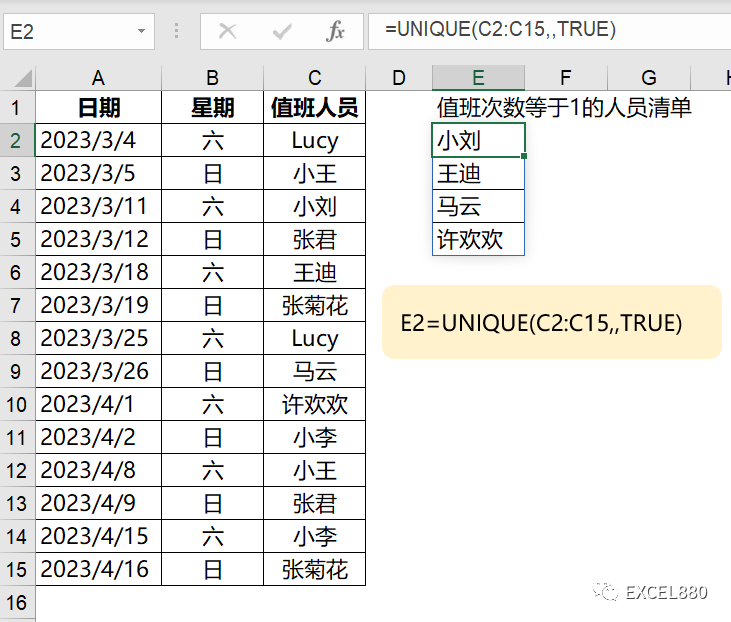Excel880笔记：OFFSET不支持隔行或隔列的数组输出，但可以用于求和。

=OFFSET(A3,,{2,4,6})

OFFSET的数组运用无法以数组形式体现结果。

=SUM(OFFSET(A3,,{2,4,6}))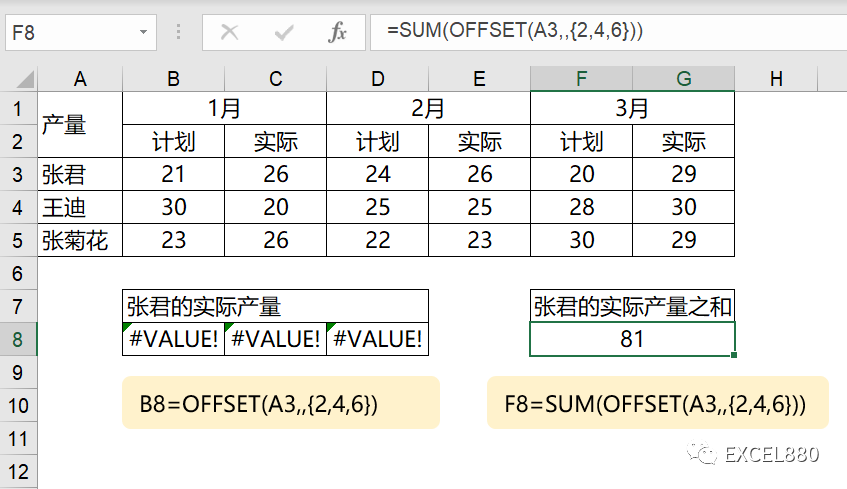Excel880笔记：数组参数也是有方向的，逗号表示横向，分号表示纵向。

=VLOOKUP(“小王”,A3:G9,{3,5,7},0)

=VLOOKUP(“小王”,A3:G9,{3;5;7},0)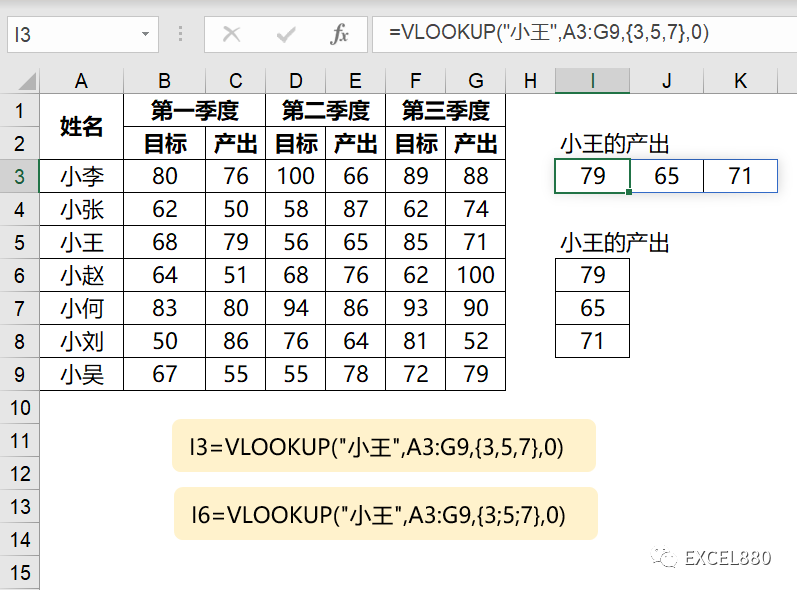Excel880笔记：VLOOKUP/XLOOKUP函数返回的空值变成0

=VLOOKUP(D2,A:B,2,0)

=VLOOKUP(D7,A:B,2,0)&””